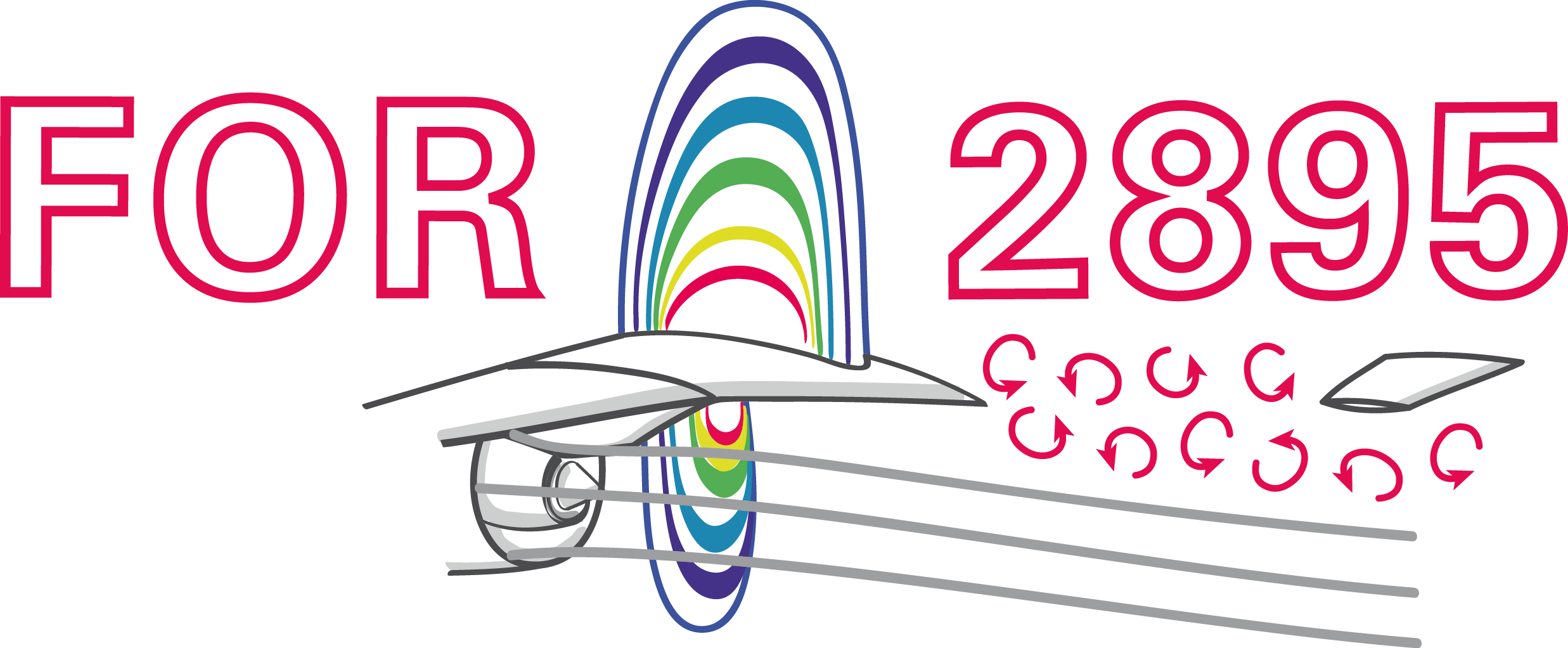### Active flow control via deep reinforcement learning implemented in OpenFOAM

Andre Weiner, Fabian Gabriel, Darshan Thummar, Richard Semaan
TU Braunschweig, Institute of Fluid Mechanics

## Outline

1. Combining ML and CFD
2. Deep reinforcement learning (DRL)
• Reinforcement learning (RL) basics
• Proximal policy optimization (PPO)
• DRL with OpenFOAM and PyTorch
3. Where to go from here

## Combining ML and CFD why and how

### Why combine CFD and ML?

CFD

• produces large amounts of complex data
• requires data or representations thereof

ML

• finds patterns in data
• creates useful representations of data

### What is data?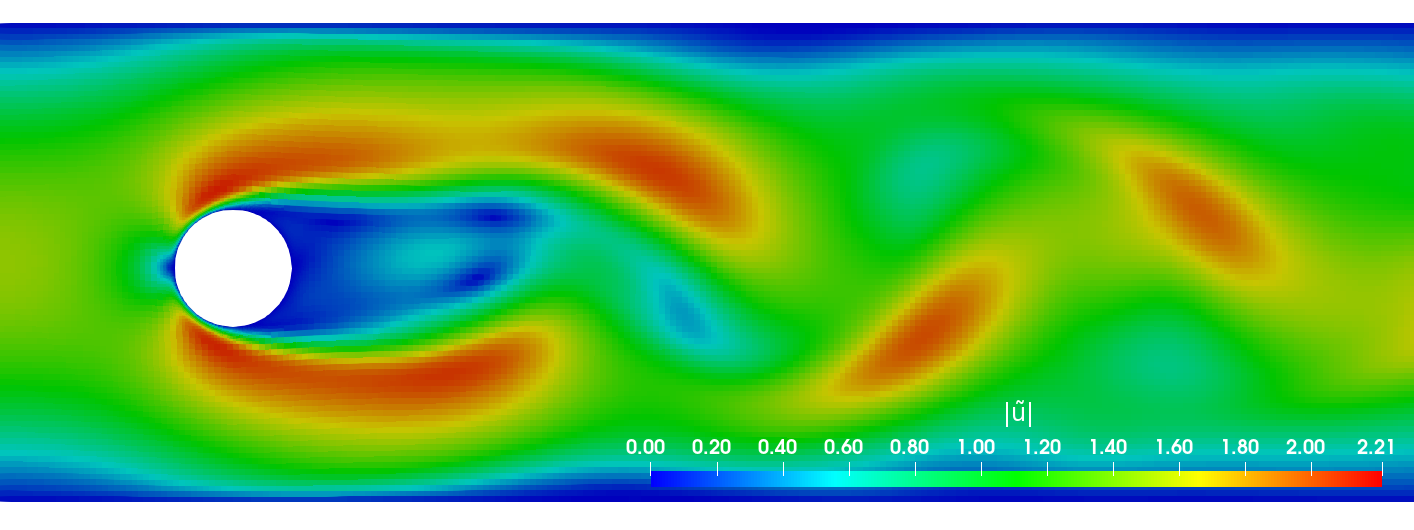primary data: scalar/vector fields, boundary fields, integral values


# log.rhoPimpleFoam
Courant Number mean: 0.020065182 max: 0.77497916
deltaT = 6.4813615e-07
Time = 1.22219e-06

PIMPLE: iteration 1
diagonal:  Solving for rho, Initial residual = 0, Final residual = 0, No Iterations 0
DILUPBiCGStab:  Solving for Ux, Initial residual = 0.0034181127, Final residual = 6.0056507e-05, No Iterations 1
DILUPBiCGStab:  Solving for Uy, Initial residual = 0.0052004883, Final residual = 0.00012352706, No Iterations 1
DILUPBiCGStab:  Solving for e, Initial residual = 0.06200185, Final residual = 0.0014223046, No Iterations 1
limitTemperature limitT Lower limited 0 (0%) of cells
limitTemperature limitT Upper limited 0 (0%) of cells
limitTemperature limitT Unlimited Tmax 329.54945
Unlimited Tmin 280.90821


Checking geometry...
...
Mesh has 2 solution (non-empty) directions (1 1 0)
All edges aligned with or perpendicular to non-empty directions.
Boundary openness (1.4469362e-19 3.3639901e-21 -2.058499e-13) OK.
Max cell openness = 2.4668495e-16 OK.
Max aspect ratio = 3.0216602 OK.
Minimum face area = 7.0705331e-08. Maximum face area = 0.00033983685.  Face area magnitudes OK.
Min volume = 1.2975842e-10. Max volume = 6.2366859e-07.  Total volume = 0.0017254212.  Cell volumes OK.
Mesh non-orthogonality Max: 60.489216 average: 4.0292071
Non-orthogonality check OK.
Face pyramids OK.
Max skewness = 1.1453509 OK.
Coupled point location match (average 0) OK.



secondary data: log files, input dictionaries, mesh quality metrics, ...

### Examples for data-driven workflowsExample: creating a surrogate or reduced-order model based on numerical data.Example: creating a space and time dependent boundary condition based on numerical or experimental data.Example: creating closure models based on numerical data.Example: active flow control or shape optimization.

### Supervised learningCreating a mapping from features to labels based on examples.

Species transport around a rising bubble:

1. simulation of two-phase flow dynamics
(specialized tool, not OpenFOAM)
2. ML models describing interface motion
3. single phase simulations with species transport

github.com/AndreWeiner/sgs_model_test_transient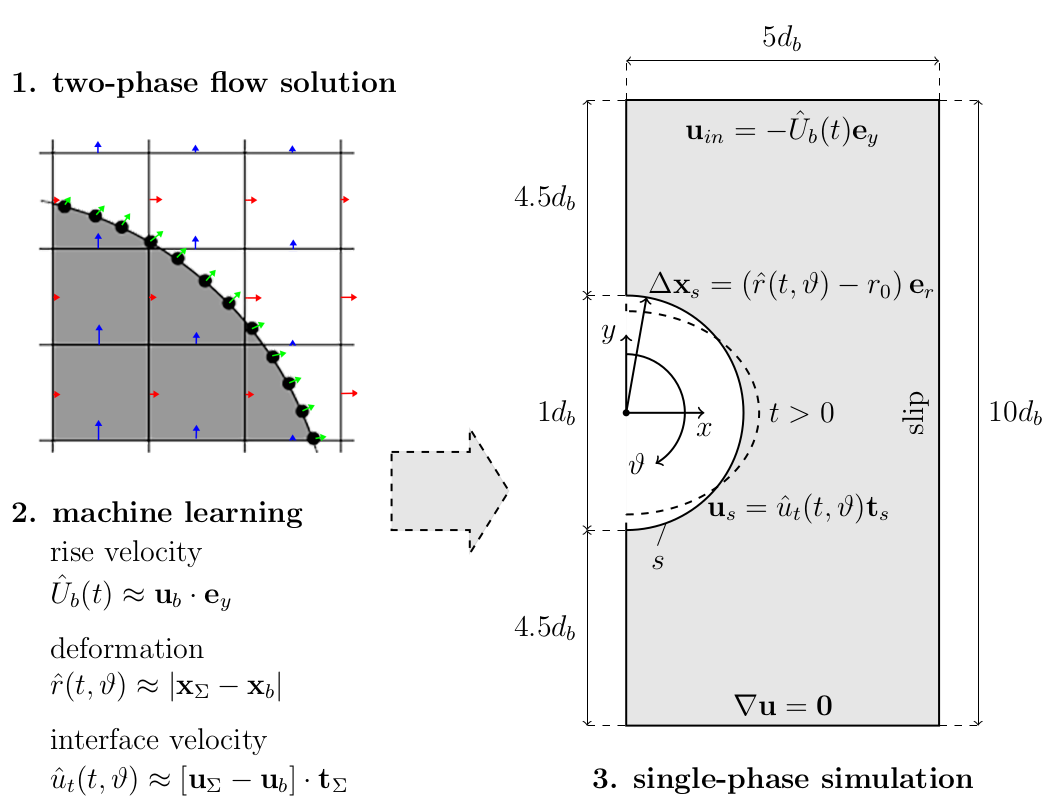Mesh motion and zoom view of concentration boundary layer for $Re=569$ and $Sc=100$.

### Unsupervised learningFinding patterns in unlabeled data.

Dynamic mode decomposition (DMD):

• transient flow is decomposed into
• spatial modes (const. in time)
• dynamic behavior (freq. + amplitude)
• STDMD function object
• flowTorch library

github.com/AndreWeiner/naca0012_shock_buffet

Slice of local Mach number $Ma$, 3D, $\alpha=4^\circ$.

DMD buffet mode recon. based on slice of $u_x$.

### What if my problem does not fit into these categories?

• break down problem into smaller junks
• mathematical, physical, numerical modeling
• combination of ML techniques

## Reinforcement learning basics

### (Deep) Reinforcement learningCreate an intelligent agent that learns to map states to actions such that cumulative rewards are maximized.

Experience tuple:

$$\left\{ S_t, A_t, R_{t+1}, S_{t+1}\right\}$$

Trajectory:

$\left\{S_0, A_0, R_1, S_1\right\}$
$\left\{S_1, A_1, R_2, S_3\right\}$
$\left\{ ...\right\}$

Long-term consequences:

$$G_t = \sum\limits_{l=0}^{N_t-t} \gamma^l R_{t+l}$$

• $t$ - control time step
• $G_t$ - discounted return
• $\gamma$ - discount factor, typically $\gamma=0.99$
• $N_t$ - number of control steps

## Proximal policy optimization

### Why PPO?

• continuous and discrete actions spaces
• relatively simple implementation
• sample efficient
• ...

Refer to R. Paris et al. 2021 and the references therein for similar works employing PPO.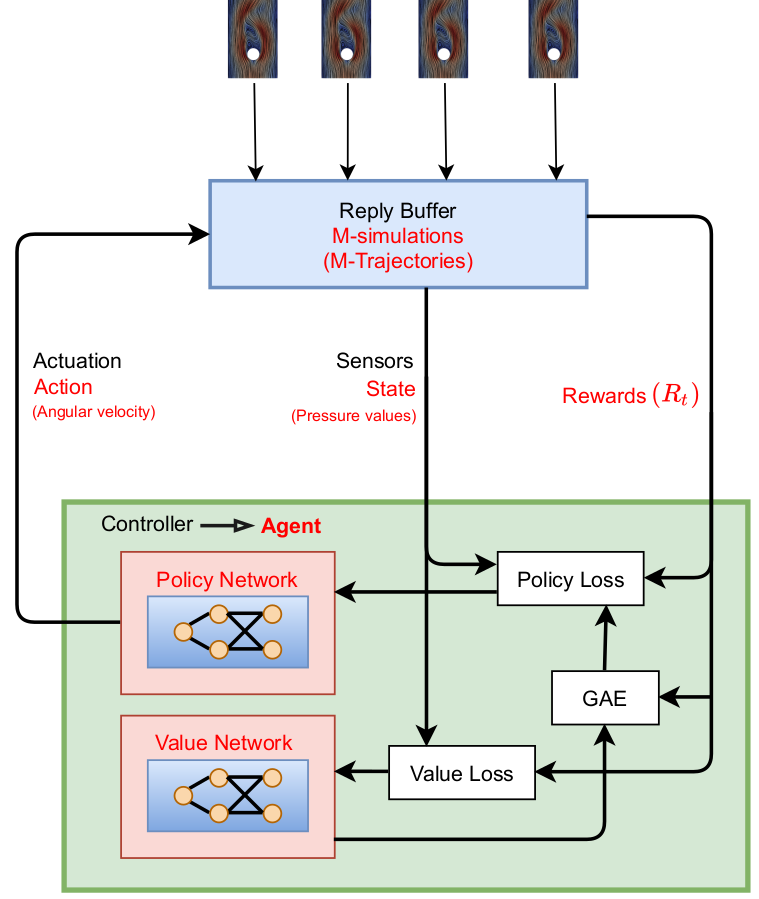Proximal policy optimization (PPO) workflow (GAE - generalized advantage estimate).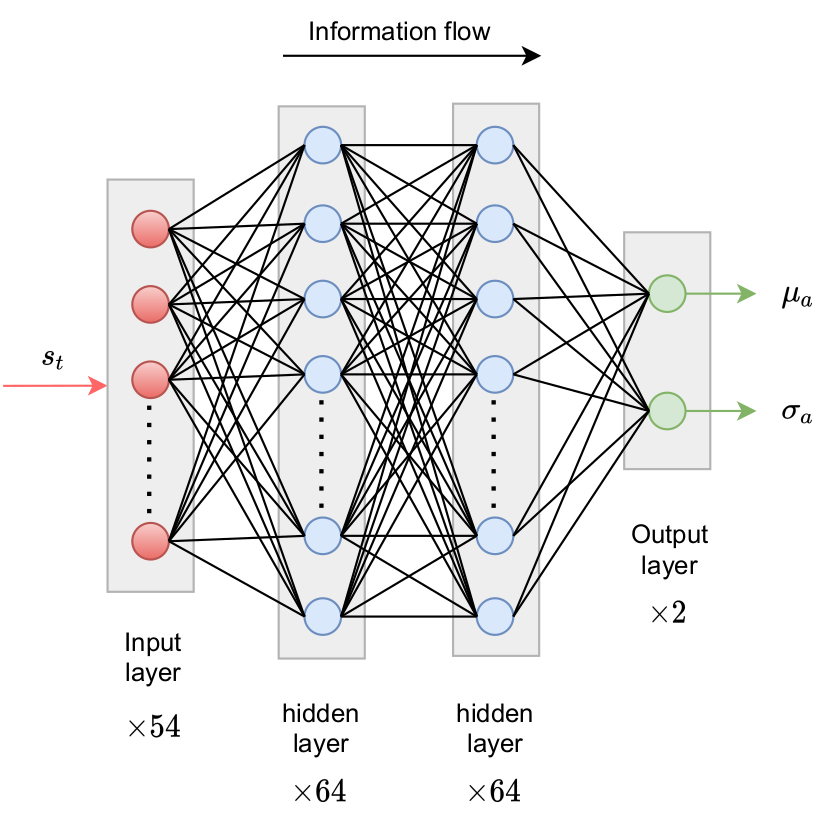Policy network predicts probability density function(s) for action(s).Comparison of Gauss and Beta distribution.

learning what to expect in a given state - value function loss

$$L_V = \frac{1}{N_\tau N_t} \sum\limits_{\tau = 1}^{N_\tau}\sum\limits_{t = 1}^{N_t} \left( V(s_t^\tau) - G_t^\tau \right)^2$$

• $\tau$ - trajectory (single simulation)
• $s_t$ - state/observation (pressure)
• $V$ - parametrized value function
• clipping not included

Was the selected action a good one?

$$\delta_t = R_t + \gamma V(s_{t+1}) - V(s_t)$$ $$A_t^{GAE} = \sum\limits_{l=0}^{N_t-t} (\gamma \lambda)^l \delta_{t+l}$$

• $\delta_t$ - one-step advantage estimate
• $A_t^{GAE}$ - generalized advantage estimate
• $\lambda$ - smoothing parameter

make good actions more likely - policy objective function

$$J_\pi = \frac{1}{N_\tau N_t} \sum\limits_{\tau = 1}^{N_\tau}\sum\limits_{t = 1}^{N_t} \left( \frac{\pi(a_t|s_t)}{\pi^{old}(a_t|s_t)} A^{GAE,\tau}_t\right)$$

• $\pi$ - current policy
• $\pi^{old}$ - old policy (previous episode)
• clipping and entropy not included
• $J_\pi$ is maximized

## DRL with OpenFOAM and PyTorch

Active control of the flow past a cylinder

Flow past a circular cylinder at $Re=100$.

Things we might be interested in:

• reduce drag and lift forces
• mitigate extreme events
• maximize mixing in the wake
• ...

Can we reduce drag and lift forces?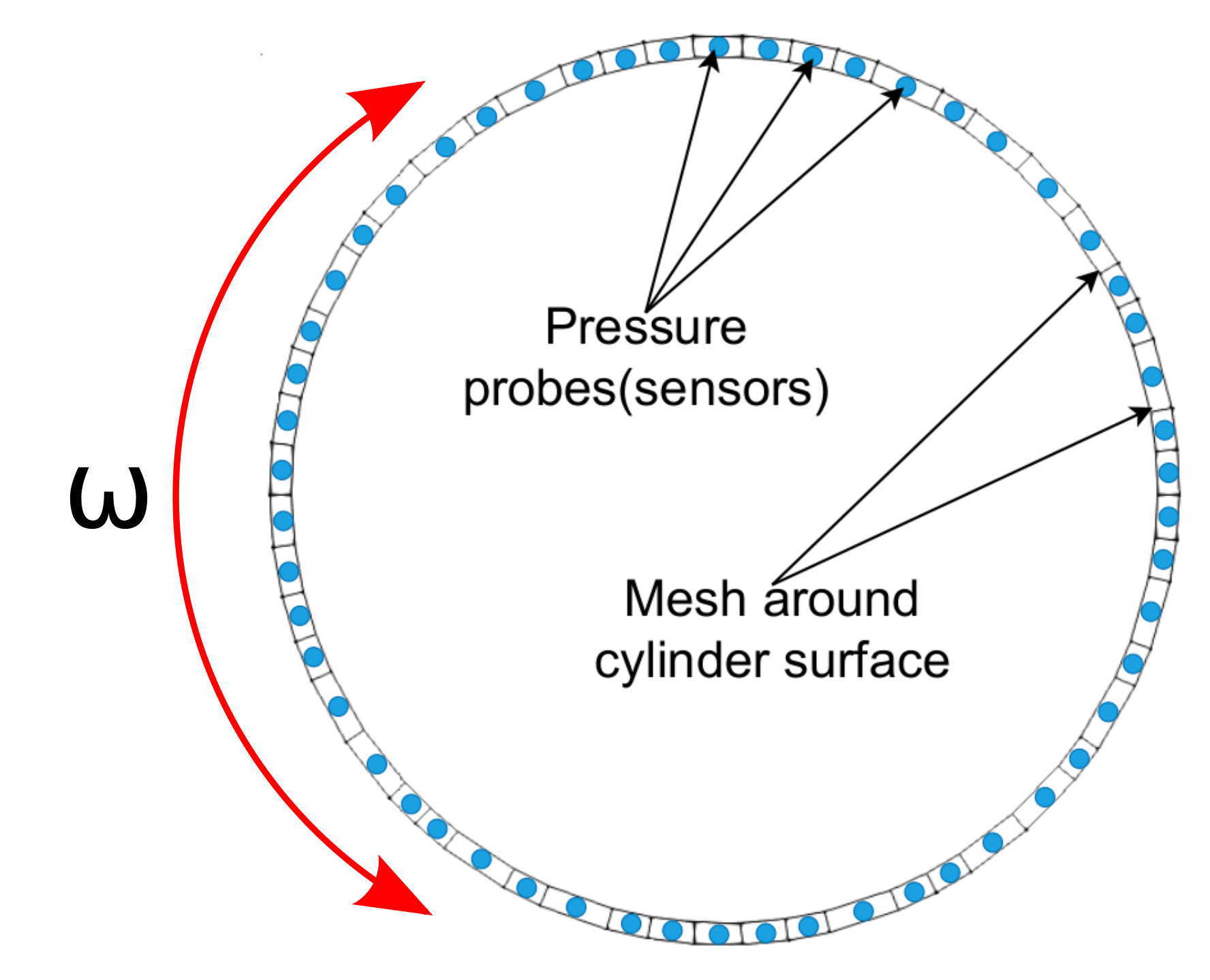rewards - expressing the goal

$$R_t = r_0 - \left( r_1 c_D + r_2 |c_L| \right)$$

• $c_D$ - drag coefficient
• $c_L$ - lift coefficient
• $r_i$ - constants

Python/PyTorch

1. create policy and value networks
2. fill trajectory buffer (run simulations)
3. update policy and value networks
4. go back to 1. until converged

Implementation follows closely chapter 12 of Miguel Morales's Grokking Deep Reinforcement Learning

C++/OpenFOAM/PyTorch

2. sample and apply action
3. write trajectory (experience tuples)

Boundary condition defined in 0/U


cylinder
{
type            agentRotatingWallVelocity;
// center of cylinder
origin          (0.2 0.2 0.0);
// axis of rotation; normal to 2D domain
axis            (0 0 1);
// name of the policy network; must be a torchscript file
policy          "policy.pt";
// when to start controlling
startTime       0.01;
// how often to evaluate policy
interval        20;
// if true, the angular velocity is sampled from a Gaussian distribution
// if false, the mean value predicted by the policy is used
train           true;
// maximum allowed angular velocity
absOmegaMax     0.05;
}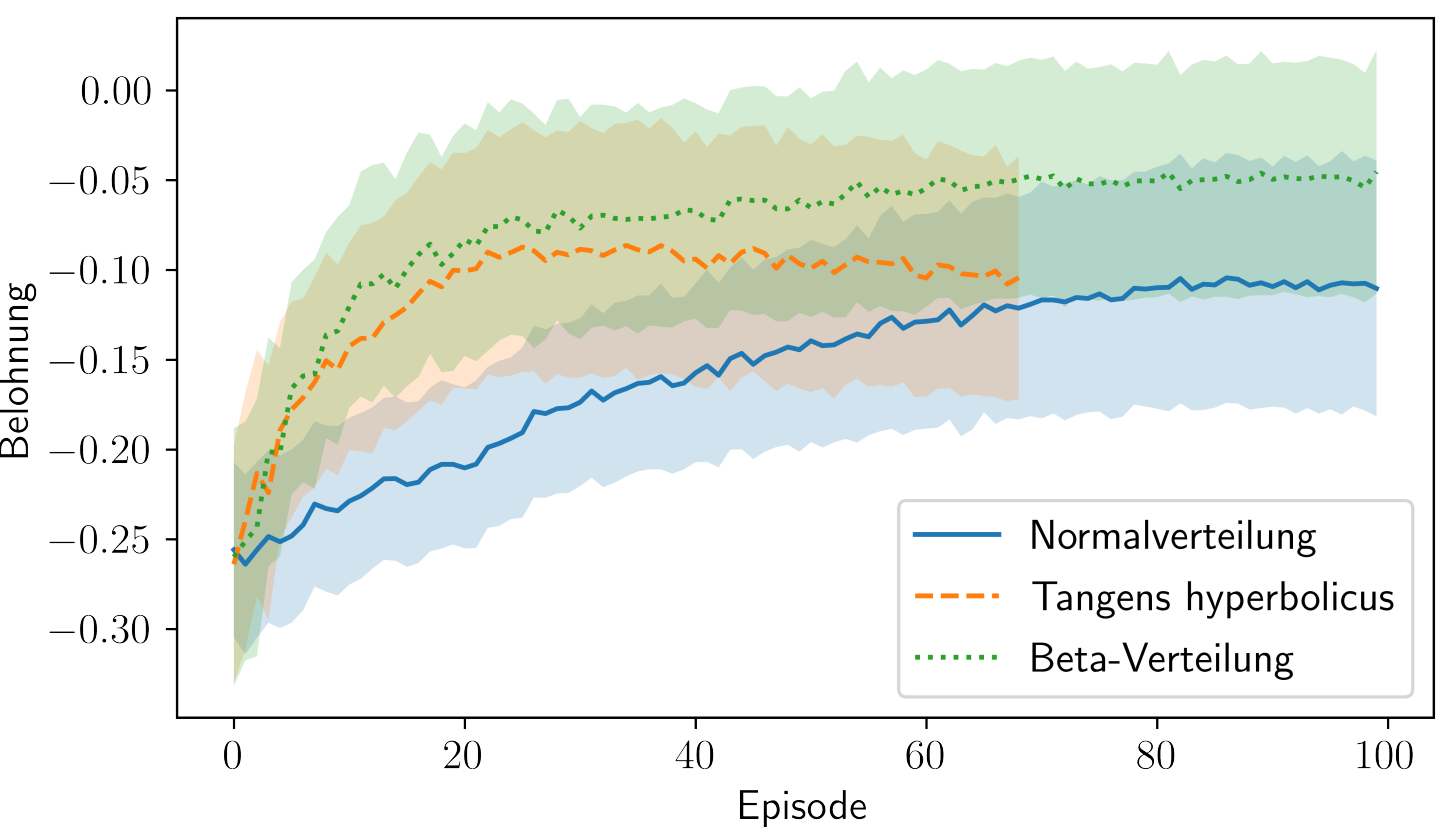Cumulative rewards vs. episodes for various distributions.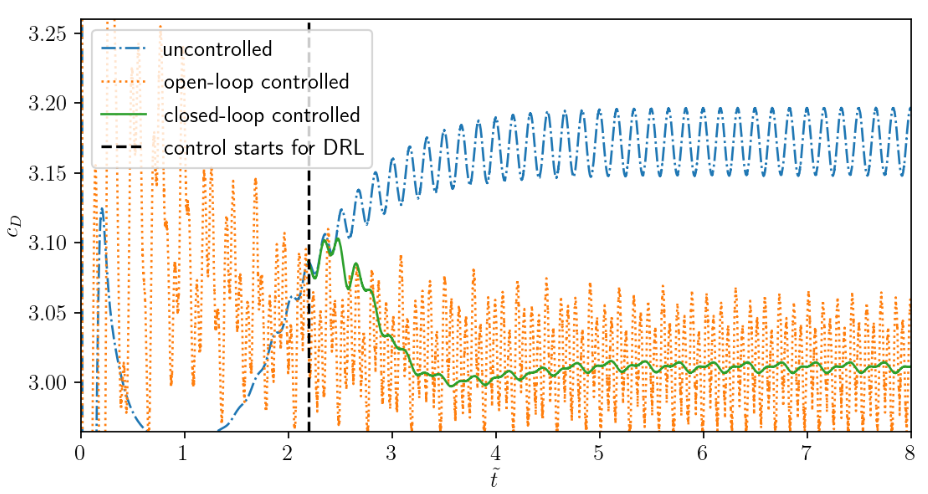Comparison of uncontrolled, open-loop controlled, and closed-loop controlled drag.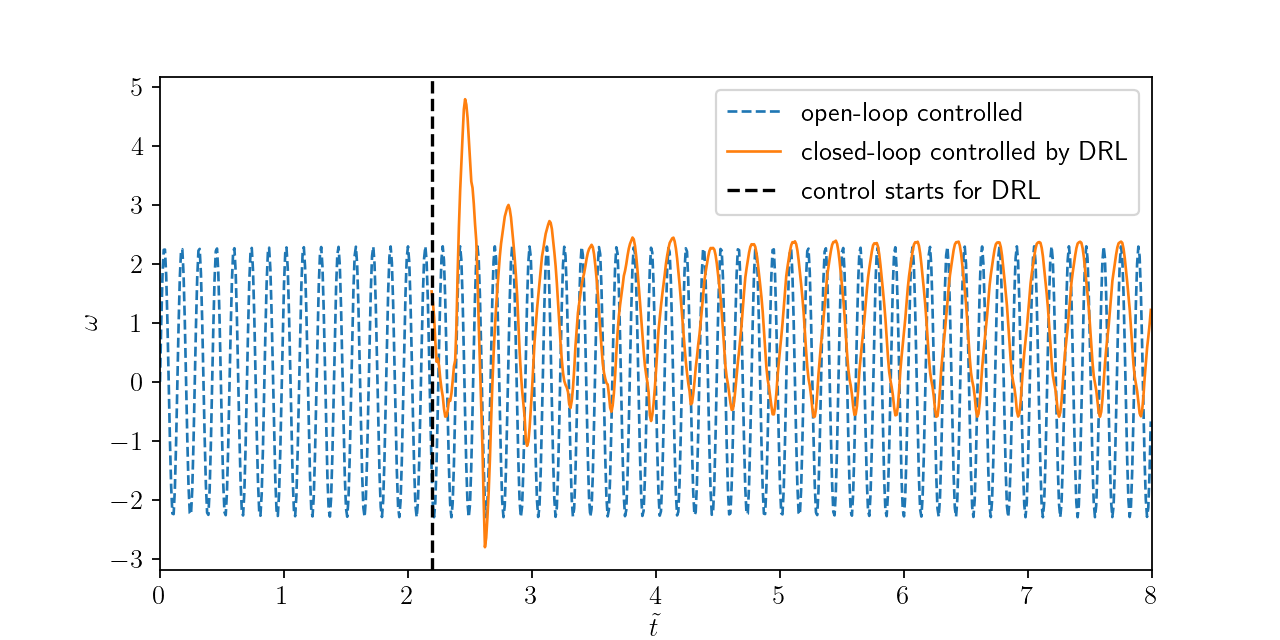Angular velocity for open and closed-loop control.

### How robust is the controller?

1. training with steady inlet velocity
$Re=\{100, 200, 400 \}$
2. test with unsteady inlet velocity
$Re(t)= 250 + 150\mathrm{sin}(\pi t)$

Variable inlet velocity/Reynolds number $Re(t) = 250 + 150\mathrm{sin}(\pi t)$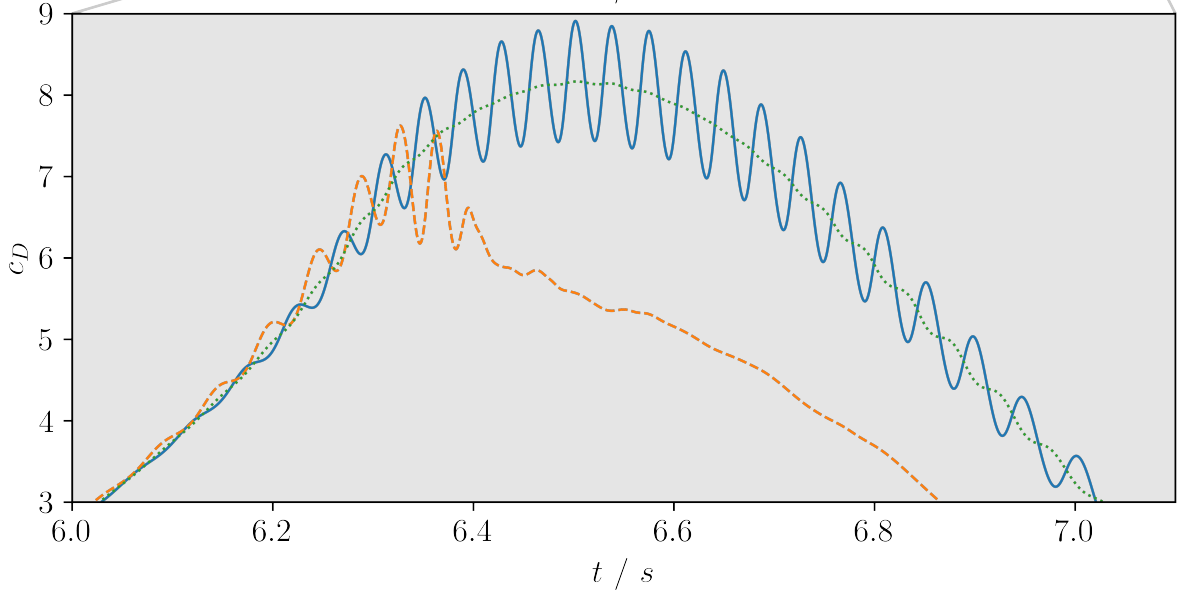Drag coefficient for transient inlet velocity: uncontrolled and controlled.

## Where to go from here

### Lecture on ML in CFD

• covers all types of ML
• freely available on Github
• work in progress

# THE END

### Thank you for you attention!